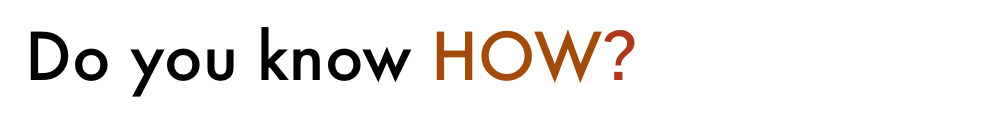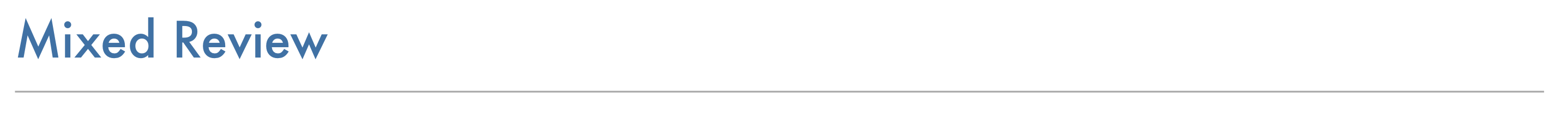Geometry 6-9 Complete Lesson: Proofs Using Coordinate Geometry
starstarstarstarstarstarstarstarstarstar
by Matthew Richardson
| 16 Questions
Note from the author:
A complete formative lesson with embedded slideshow, mini lecture screencasts, checks for understanding, practice items, mixed review, and reflection. I create these assignments to supplement each lesson of Pearson's Common Core Edition Algebra 1, Algebra 2, and Geometry courses. See also mathquest.net and twitter.com/mathquestEDU.
1
10
Solve It! The coordinates of three vertices of a rectangle are (-2a, 0), (2a, 0), and (2a, 2b). A diagonal joins one of these points with the fourth vertex. What are the coordinates of the midpoint of the diagonal? Justify your answer.2
2
10
Problem 1 Got It? What is the advantage of using coordinates O(0, 0), E(0, 2b), and F(2a, 0) rather than O(0, 0), E(0, b), and F(a, 0)?3
3
40
Problem 2 Got It? Write a coordinate proof of the Triangle Midsegment Theorem (Theorem 5-1).4
50
Coordinate Proof: Use coordinate geometry to prove that the diagonals of a rectangle are congruent.6
6
10
Error Analysis: Your classmate places a trapezoid on the coordinate plane. What is the error?7
10
Review Lesson 6-8: A rectangle ABCD is centered at the origin with A(-a, b). Without using any new variables, what are the coordinates of point C?
(a, b)
(a, -b)
(-a, -b)
(-a, b)8
9
10
8
5
Review Lessons 4-2, 4-3, and 4-4: Which theorem or postulate would you use, along with corresponding parts of congruent triangles (CPCTC), to prove the congruence?
SSS
SAS
ASA
AAS
9
5
Review Lessons 4-2, 4-3, and 4-4: Which theorem or postulate would you use, along with CPCTC, to prove the congruence?
SSS
SAS
ASA
AAS
10
5
Review Lessons 4-2, 4-3, and 4-4: Which theorem or postulate would you use, along with CPCTC, to prove the congruence?
SSS
SAS
ASA
AAS
11
10
Review Algebra: Solve. Round to the nearest tenth if necessary.
Select all that apply.
x = 14
x = 12
x = 144
x = -12
12
10
Review Algebra: Solve. Round to the nearest tenth if necessary.
Select all that apply.
r = -8.1
r = -8
r = 8
r = 8.1
13
10
Review Algebra: Solve. Round to the nearest tenth if necessary.
Select all that apply.
k = -19.3
k = -16.6
k = 16.6
k = 19.314
10
Vocabulary Review: Follow the instructions to complete the items on the canvas.15
10
Vocabulary Review: Follow the instructions to complete the items on the canvas.
16
10
Reflection: Math Success